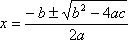• If you are citizen of an European Union member nation, you may not use this service unless you are at least 16 years old.

• You already know Dokkio is an AI-powered assistant to organize & manage your digital files & messages. Very soon, Dokkio will support Outlook as well as One Drive. Check it out today!

View

last edited by 16 years, 6 months ago

Originators: Tom and Nicole

Explanation: The quadratic formula is derived from the function y=ax2+bx+c. As long as your function can be put into general form, you can use the quadratic formula to find its roots and discriminate.The roots allow us to see where the function passes throught the x-intercept, if it does at all. The roots can either be real or complex numbers. The discriminate (b2-4ac) lets us see how many x-intercepts we're dealing with. If the discriminate is > 0 the function will have 2 real roots. If the discriminate is = 0 then you have one real root.

And if the discriminate is < 0 you have 0 real roots.

The + - in the quadratic formula is there so that you can get both possible roots.It's there because when you took the the square root of each side of the equation, it could be either positive or negitive numbers.

Sample Problem:

Question

Given the function.Create a graph,state the discriminate, and find the roots using the quadratic formula.

Solution/Graph

(need graph)

Solution/DiscriminateThe discriminate is 0 therefore, you have 1 double real root.

Solution/RootsBoth roots are 8.